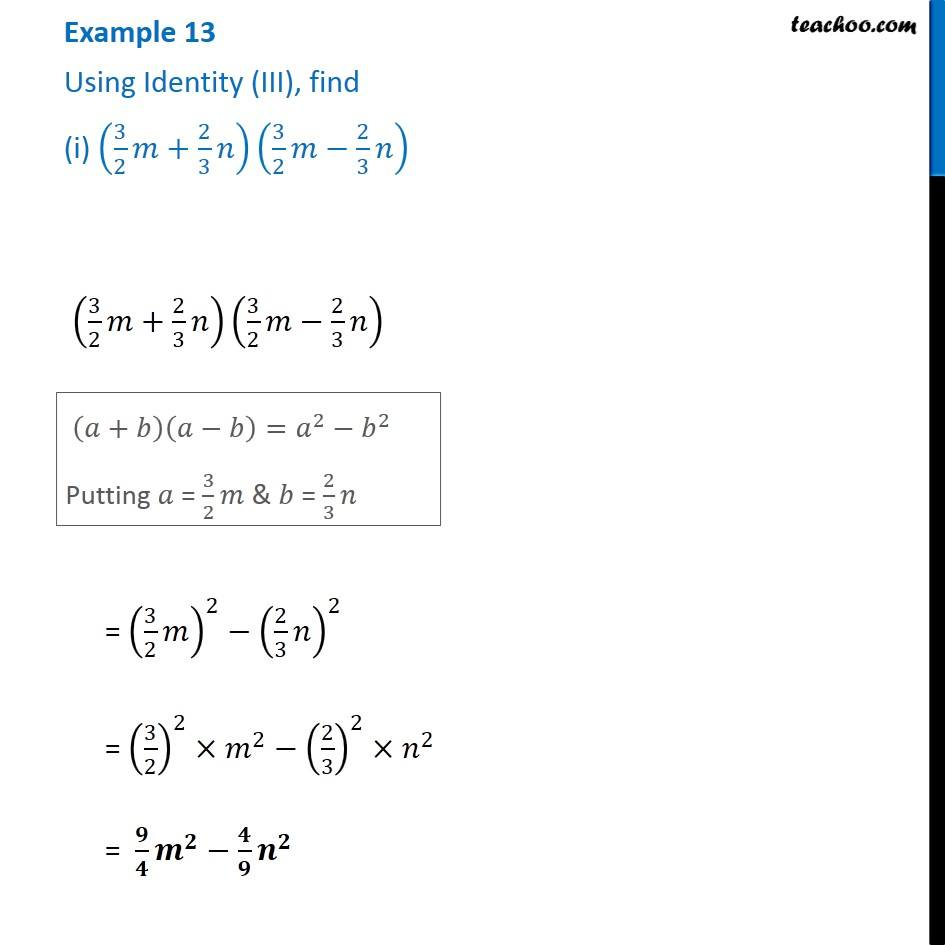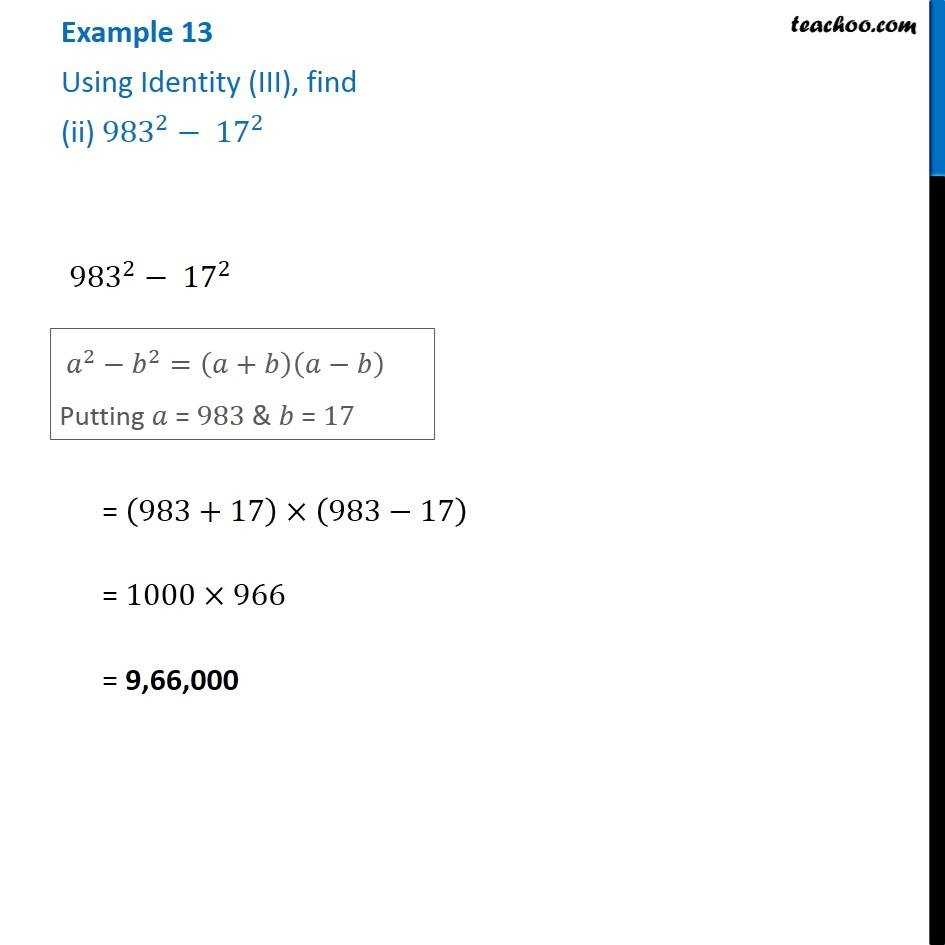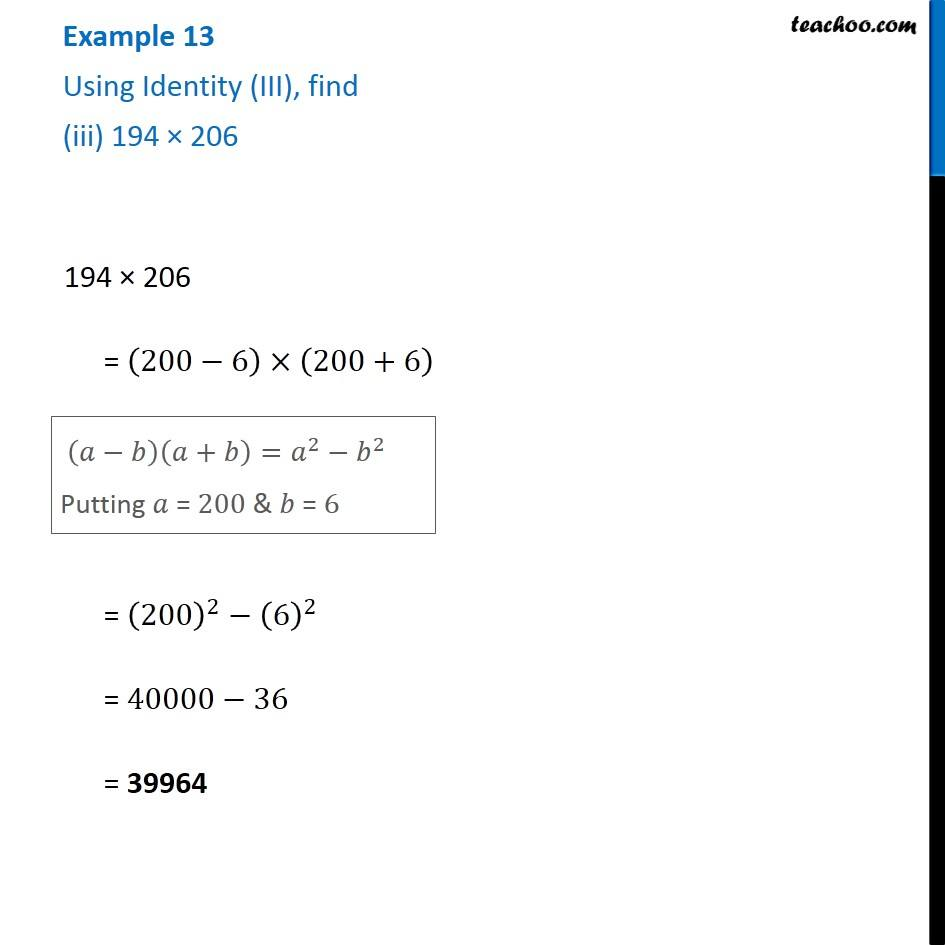1. Chapter 9 Class 8 Algebraic Expressions and Identities
2. Serial order wise
3. Examples

Transcript

Example 13 Using Identity (III), find (i) (3/2 𝑚+2/3 𝑛)(3/2 𝑚−2/3 𝑛) (3/2 𝑚+2/3 𝑛)(3/2 𝑚−2/3 𝑛) (𝑎+𝑏)(𝑎−𝑏)=𝑎^2−𝑏^2 Putting 𝑎 = 3/2 𝑚 & 𝑏 = 2/3 𝑛 = (3/2 𝑚)^2−(2/3 𝑛)^2 = (3/2)^2×𝑚^2−(2/3)^2×𝑛^2 = 𝟗/𝟒 𝒎^𝟐−𝟒/𝟗 𝒏^𝟐 Example 13 Using Identity (III), find (ii) 〖983〗^2− 〖17〗^2 〖983〗^2− 〖17〗^2 𝑎^2−𝑏^2=(𝑎+𝑏)(𝑎−𝑏) Putting 𝑎 = 983 & 𝑏 = 17 = (983+17)×(983−17) = 1000×966 = 9,66,000 Example 13 Using Identity (III), find (iii) 194 × 206 194 × 206 = (200−6)×(200+6) 194 × 206 = (200−6)×(200+6) = (200)^2−(6)^2 = 40000−36 = 39964

Examples

About the AuthorDavneet Singh
Davneet Singh is a graduate from Indian Institute of Technology, Kanpur. He has been teaching from the past 10 years. He provides courses for Maths and Science at Teachoo.